Test: Continuity Equation

# Test: Continuity Equation

Test Description

## 10 Questions MCQ Test Fluid Mechanics for Civil Engineering | Test: Continuity Equation

Test: Continuity Equation for Civil Engineering (CE) 2023 is part of Fluid Mechanics for Civil Engineering preparation. The Test: Continuity Equation questions and answers have been prepared according to the Civil Engineering (CE) exam syllabus.The Test: Continuity Equation MCQs are made for Civil Engineering (CE) 2023 Exam. Find important definitions, questions, notes, meanings, examples, exercises, MCQs and online tests for Test: Continuity Equation below.
Solutions of Test: Continuity Equation questions in English are available as part of our Fluid Mechanics for Civil Engineering for Civil Engineering (CE) & Test: Continuity Equation solutions in Hindi for Fluid Mechanics for Civil Engineering course. Download more important topics, notes, lectures and mock test series for Civil Engineering (CE) Exam by signing up for free. Attempt Test: Continuity Equation | 10 questions in 30 minutes | Mock test for Civil Engineering (CE) preparation | Free important questions MCQ to study Fluid Mechanics for Civil Engineering for Civil Engineering (CE) Exam | Download free PDF with solutions
 1 Crore+ students have signed up on EduRev. Have you?
Test: Continuity Equation - Question 1

### If a liquid enters a pipe of diameter d with a velocity v, what will it’s velocity at the exit if the diameter reduces to 0.5d?

Detailed Solution for Test: Continuity Equation - Question 1

According to the Continuity Equation,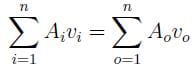where a represents flow area, v represents flow velocity, i is for inlet conditions and o is for outlet conditions.
If,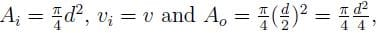vo = 4v

Test: Continuity Equation - Question 2

### Two pipes, each of diameter d, converge to form a pipe of diameter D. What should be the relation between d and D such that the flow velocity in the third pipe becomes double of that in each of the two pipes?

Detailed Solution for Test: Continuity Equation - Question 2

According to the Continuity Equation,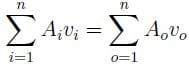where a represents flow area, v represents flow velocity, i is for inlet conditions and o is for outlet conditions. Thus,
A1v1 + A2v2 = Av
d2v + d2v = D2v
D = d.

Test: Continuity Equation - Question 3

### In a two dimensional flow, the component of the velocity along the X-axis and the Y-axis are u = ax2 + bxy + cy2 and v = cxy. What should be the condition for the flow field to be continuous?

Detailed Solution for Test: Continuity Equation - Question 3

According to the condition for continuity,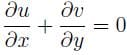2ax + cx = 0
2a + c = 0.

Test: Continuity Equation - Question 4

In a two dimensional flow, the component of the velocity along the X-axis and the Y-axis are u = ax2 + bxy and v = bxy + ay2. The condition for the flow field to be continuous is

Detailed Solution for Test: Continuity Equation - Question 4

The condition for the flow field to be continuous is: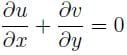2ax + by + 2ay + bx = 0
x + y = 0
Hence, the condition for the flow field to be continuous is independent of the constants (a; b) and dependent only on the variables (x; y).

Test: Continuity Equation - Question 5

In a water supply system, water flows in from pipes 1 and 2 and goes out from pipes 3 and 4 as shown. If all the pipes have the same diameter, which of the following must be correct?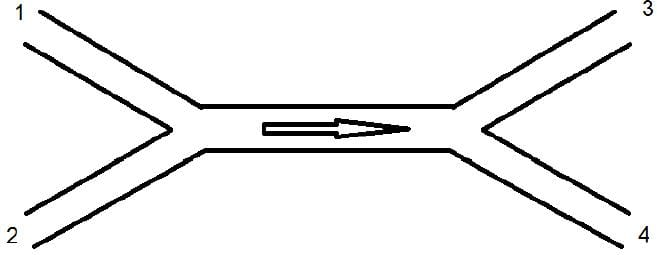Detailed Solution for Test: Continuity Equation - Question 5

According to the Continuity Equation,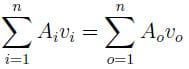where a represents flow area, v represents flow velocity, i is for inlet conditions and o is for outlet conditions.
A1v1 + A2v2 = A3v3 + A4v4
Since d1 = d2 = d3 = d4, v1 + v2 = v3 + v4.

Test: Continuity Equation - Question 6

The continuity equation is based on the principle of

Detailed Solution for Test: Continuity Equation - Question 6

According to the Continuity Equation, if no fluid is added or removed from the pipe in any length then the mass passing across different sections shall be the same. This is in accordance with the principle of conservation of mass which states that matter can neither be created nor be destroyed.

Test: Continuity Equation - Question 7

Two pipes of diameters d1 and d2 converge to form a pipe of diameter 2d. If the liquid flows with a velocity of v1 and v2 in the two pipes, what will be the flow velocity in the third pipe?

Detailed Solution for Test: Continuity Equation - Question 7

According to the Continuity Equation,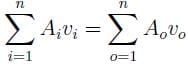where a represents flow area, v represents flow velocity, i is for inlet conditions and o is for outlet conditions. Thus,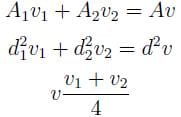Test: Continuity Equation - Question 8

St. venant equations represent

Detailed Solution for Test: Continuity Equation - Question 8

St. Venant Equations:
1. Continuity equation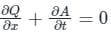2. Momentum Equation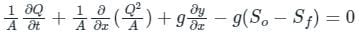Assumptions for St. Venant Equations

• Flow is one-dimensional
• Hydrostatic pressure prevails and vertical accelerations are negligible
• Streamline curvature is small.
• Bottom slope of the channel is small.
• Manning’s equation is used to describe resistance effects
• The fluid is incompressible

Hence, St. venant equations represent continuity and momentum equations.

Test: Continuity Equation - Question 9

A fluid flowing through a pipe of diameter 450 mm with velocity 3 m/s is divided into two pipes of diameters 300 mm and 200 mm. The velocity of flow in 300 mm diameter pipe is 2.5 m/s, then the velocity of flow through 200 mm diameter pipe will be

Detailed Solution for Test: Continuity Equation - Question 9

Concept:
According to continuity, the liquid flow rate will be conserved.
Q1 = Q2 + Q3
In a pipe, flow rate is given by
Q = AV
Calculation:
Given V1 = 3 m/s, D1 = 450 mm, V2 = 2.5 m/s, D2 = 300 mm, D3 = 200 mm;
From continuity,
D12 V1 = D22 V2 + D32 V3
⇒ 4502 × 3 = 3002 × 2.5 + 2002 × V3
⇒ V3 = 9.56 m/s

*Answer can only contain numeric values
Test: Continuity Equation - Question 10

A channel of width 450 mm branches into two sub-channels having width 300 mm and 200 mm as shown in figure. If the volumetric flow rate (taking unit depth) of an incompressible flow through the main channel is 0.9 m3/s and the velocity in the sub-channel of width 200 mm is 3 m/s, the velocity in the sub-channel of width 300 mm is ______m/s
Assume both inlet and otlet to be at the same elevation.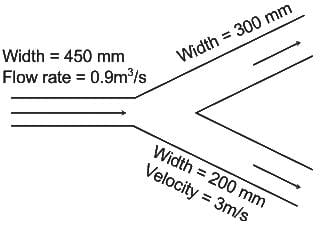Detailed Solution for Test: Continuity Equation - Question 10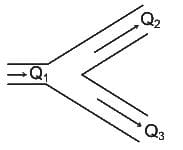Concept:
The principle used here is Conservation of mass
∴ Q1 = Q2 + Q3
Calculation:
Q1 = Q2 + Q3
A1V1 = A2V+ A3V3
⇒ 0.9 = 300 × 10-3 × V2 + 200 × 10-3 × 3
⇒ 0.9 = 0.3 V2 + 0.6
⇒ V2 = 1 m/s

## Fluid Mechanics for Civil Engineering

43 videos|73 docs|87 tests
Information about Test: Continuity Equation Page
In this test you can find the Exam questions for Test: Continuity Equation solved & explained in the simplest way possible. Besides giving Questions and answers for Test: Continuity Equation, EduRev gives you an ample number of Online tests for practice

## Fluid Mechanics for Civil Engineering

43 videos|73 docs|87 tests(Scan QR code)